# Excel LEFT function with formula examples

The tutorial shows how to use the LEFT function in Excel to get a substring from the beginning of the text string, extract text before a certain character, force a Left formula to return a number, and more.

Among many different functions that Microsoft Excel provides for manipulating text data, LEFT is one of the most widely used. As its name suggests, the function allows you to extract a certain number of characters starting at the left side of a text string. However, Excel LEFT is capable of much more than its pure essence. In this tutorial, you will find a couple of basic Left formulas to understand the syntax, and then I will show you a few ways in which you can take the Excel LEFT function well beyond its basic usage.

## Excel LEFT function - syntax

The LEFT function in Excel returns the specified number of characters (substring) from the start of a string.

The syntax of the LEFT function is as follows:

LEFT(text, [num_chars])

Where:

• Text (required) is the text string from which you want to extract a substring. Usually it is supplied as a reference to the cell containing the text.
• Num_chars (optional) - the number of characters to extract, starting on the left side of the string.
• If num_chars is omitted, it defaults to 1, meaning that a Left formula will return 1 character.
• If num_chars is greater than the total length of text, a Left formula will return all of text.

For example, to extract the first 3 characters from the text in cell A2, use this formula:

`=LEFT(A2, 3)`

The following screenshot shows the result: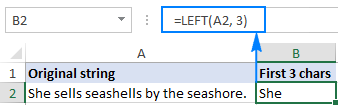Important note! LEFT belongs to the category of Text functions, therefore the result of a Left formula is always a text string, even if the original value from which you extract characters is a number. If you are working with a numeric dataset and want the LEFT function to return a number, use it in conjunction with the VALUE function as demonstrated in this example.

## How to use LEFT function in Excel - formula examples

Apart from extracting text from the left of a string, what else can the LEFT function do? The following examples show how you can use LEFT in combination with other Excel functions to solve more complex tasks.

### How to extract a substring before a certain character

In some cases, you may need to extract the part of the text string that precedes a specific character. For example, you may want to pull the first names from a column of full names or get the country codes from a column of phone numbers. The problem is that each name and each code contains a different number of characters, and therefore you cannot simply supply a predefined number to the num_chars argument of your Left formula like we did in the above example.

If the first and last names are separated by a space, the problem boils down to working out the position of the space character in a string, which can be easily done by using either SEARCH or FIND function.

Supposing the full name is in cell A2, the position of the space is returned by this simple formula: SEARCH(" ",A2)). And now, you embed this formula in the num_chars argument of the LEFT function:

`=LEFT(A2, SEARCH(" ", A2))`

To improve the formula a bit further, get rid of the trailing space by subtracting 1 from the Search formula result (not visible in cells, trailing spaces may cause many problems especially if you plan to use the extracted names in other formulas):

`=LEFT(A2, SEARCH(" ", A2)-1)`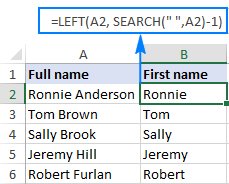In the same fashion, you can extract the country codes from a column of telephone numbers. The only difference is that you use the Search function to find out the position of the first hyphen ("-") rather than a space:

`=LEFT(A2, SEARCH("-", A2)-1)`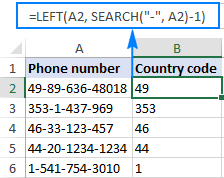Wrapping up, you can use this generic formula to get a substring that precedes any other character:

LEFT(string, SEARCH(character, string) - 1)

### How to remove the last N characters from a string

You already know how to use the Excel LEFT function to get a substring from the start of a text string. But sometimes you may want to do something different - remove a certain number of characters from the end of the string and pull the rest of the string into another cell. For this, use the LEFT function in combination with LEN, like this:

LEFT(string, LEN(string) - number_of_chars_to_remove)

The formula works with this logic: the LEN function gets the total number of characters in a string, then you subtract the number of unwanted characters from the total length, and have the LEFT function return the remaining characters.

For example, to remove the last 7 characters from text in A2, use this formula:

`=LEFT(A2, LEN(A2)-7)`

As shown in the screenshot below, the formula successfully cuts off the " - ToDo" postfix (4 letters, a hyphen and 2 spaces) from the text strings in column A.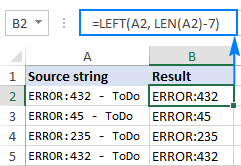### How to force the LEFT function to return a number

As you already know, the Excel LEFT function always returns text, even when you are pulling a few first digits from a number. What it means to you is that you won't be able to use the results of your Left formulas in calculations or in other Excel functions that operate on numbers.

So, how do you make Excel LEFT to output a number rather than a text string? Simply by wrapping it in the VALUE function, which is designed to convert a string representing a number to a number, like this: `VALUE(LEFT())`

For example, to extract the first 2 characters from the string in A2 and convert the output into numbers, use this formula:

`=VALUE(LEFT(A2,2))`

The result will look something similar to this: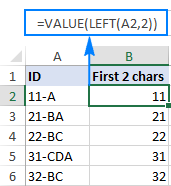As you can see in the screenshot above, the numbers in column B obtained with a Value Left formula are right-alighted in cells, as opposed to left-aligned text in column A. Since Excel recognizes the output as numbers, you are free to sum and average those values, find the min and max value, and perform any other calculations.

These are just a few of many possible uses of LEFT in Excel. To have a closer look at the formulas discussed in this tutorial, you are welcome to download the Excel LEFT function sample worksheet.

For more Left formula examples, please check out the following resources:

## Excel LEFT function not working - reasons and solutions

If the Excel LEFT function does not work properly in your worksheets, it's most likely because of one of the following reasons.

### 1. Num_chars argument is less than zero

If your Excel Left formula returns the #VALUE! error, the first thing for you to check is the value in the num_chars argument. If it's a negative number, just remove the minus sign and the error will be gone (of course, it's very unlikely that someone will put a negative number there of purpose, but to err is human :)

Most often, the VALUE error occurs when the num_chars argument is represented by another function. In this case, copy that function to another cell or select it in the formula bar and press F9 to see what it equates to. If the value is less than 0, then check the function for errors.

To better illustrate the point, let's take the Left formula we've used in the first example to extract the country phone codes: LEFT(A2, SEARCH("-", A2)-1). As you may remember, the Search function in the num_chars argument calculates the position of the first hyphen in the original string, from which we subtract 1 to remove the hyphen from the final result. If I accidentally replace -1, say, with -11, the formula would through the #VALUE error because the num_chars argument equates to a negative numbers: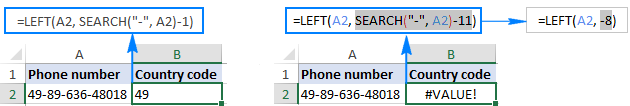### 2. Leading spaces in the original text

In case your Excel Left formula fails for no obvious reason, check the original values for leading spaces. If you have copied your data from the web or exported from another external source, many such spaces may lurk unnoticed before the text entries, and you will never know they are there until something goes wrong. The following image illustrates the problem: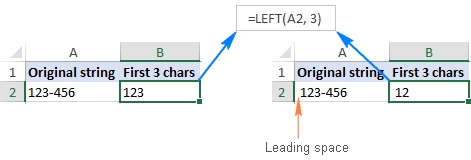To get rid of the leading spaces in your worksheets, use the Excel TRIM function or the Text Toolkit add-in.

### 3. Excel LEFT does not work with dates

If you attempt use the Excel LEFT function to get an individual part of a date (such as day, month or year), in most cases you will only retrieve the first few digits of the number that represents that date. The point is that in Microsoft Excel, all dates are stored as integers representing the number of days since January 1, 1900, which is stored as number 1 (for more information, please see Excel date format). What you see in a cell is just a visual representation of the date and its display can easily be changed by applying a different date format.

For example, if you have the date 11-Jan-2017 in cell A1 and you try to extract the day by using the formula LEFT(A1,2), the result would be 42, which is the first 2 digits of number 42746 that represents January 11, 2017 in the internal Excel system.

To extract a specific part of a date, use one of the following functions: DAY, MONTH or YEAR.

In case your dates are entered as text strings, the LEFT function will work without a hitch, as shown in the right part of the screenshot: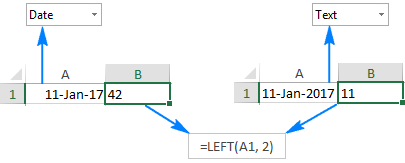This is how you use the LEFT function in Excel. I thank you for reading and hope to see you again next week.

## You may also be interested in

### 80 comments to "Excel LEFT function with formula examples"

1.Jimmy450 says:

I want to delete some letters in the right position. like the following example:

LITE TESSIAN 10P S

LITE TESSIAN 50P S +

LITE TESSIAN 40P S+

i want to remove the letters "S" , "S+" and "S +".

•Jimmy450 says:

BB SEAMUSS 100X S+
BB SEAMUSS 140X S +
LITE TESSIAN 10P S
LITE TESSIAN 50P S +
LITE TESSIAN 40P S+

i want to remove the letters "S" , "S+" and "S +".

•Alexander Trifuntov (Ablebits Team) says:

Hello!
If you want to remove specific characters from text, use this guide: Replace character(s) with nothing.
If this is not what you wanted, please describe the problem in more detail.

2.Rob Lewis says:

I've been fighting with EXACT, LEFT and data validation. I want to ensure that my spreadsheet users only enter a postcode into a particular cell. The postcodes can begin with BR, BA or GL and would be 6 or 7 characters long in total. Is there a way to enter that as a formulae into Data Validation using EXACT/LEFT ? Thanks in advance

•Alexander Trifuntov (Ablebits Team) says:

Hello!
Use the data validation tool with this formula:

=SUM(--(LEFT(A1,2)={"BR","BA","GL"}))*(LEN(A1)>5)*LEN(A1)<8

•Rob says:

Hi Alexander,

Thanks for your help with this, but I am unable to apply this formulae within custom Data Validation. I get this message.

"You may not use reference operators (such as unions, intersections, and ranges), array constants, or the LAMBDA function for Data Validation criteria"

•Alexander Trifuntov (Ablebits Team) says:

Hi!
Replace the array of values with the sum of conditions by logic OR

=((LEFT(A1,2)="BR")+(LEFT(A1,2)="BA")+(LEFT(A1,2)="GL"))*(LEN(A1)>5)*(LEN(A1)<8)

•Rob says:

Alexander - you are a STAR! Works a treat. Thank you! Now I just need to apply a slight tweak as if a user enters BS10 3FG , or GL1 3DD for example, it will return an error. If they enter BS103FG or GL13DD it accepts it. This is right, based on what you have created for me.

Would you use the SUBSTITUTE formulae to remove any space entered and would this have to go at the beginning of the formulae so the other validation happens after?

I am going to state "no spaces" on the Form next to the cell itself but know users will still put them!

Thanks again :)

•Alexander Trifuntov (Ablebits Team) says:

Hello!

=SUM(--(LEFT(A1,2)={"BR","BA","GL"}))*(LEN(A1)>5)*(LEN(A1)<8)*ISERROR(SEARCH(" ",A1))

•Rob...again says:

Yet again - exactly what I asked for - THANKS ! At the risk of pushing my luck......

I realise that the system that will read the completed form insists that postcodes with 6 digits are input with a space, and those with 7 digits do not have a space. Again, I can state this on the form but wonder if there is an extra bit I can add to the code that would take this into account, and make the user enter a space if 6, and not if 7 . Aware this is starting to become very drawn out formulae, but cannot think of a way round it that doesn't rely on users following instructions!

3.PS says:

Boy do I love this website. Can I ask how to write a formula that would count uppercase characters before the first space? I tried the following but didn't work quite right:

=LEN(LEFT(SUMPRODUCT(LEN(A1)-LEN(SUBSTITUTE(A1,CHAR(ROW(INDIRECT("65:90"))),""))))-SEARCH(" ",A1))

•Alexander Trifuntov (Ablebits Team) says:

Hello!

=SUM(--(NOT(ISNUMBER(--MID(LEFT(A1,SEARCH(" ",A1,1)), ROW(INDIRECT("\$1:\$"&(SEARCH(" ",A1,1)-1))),1)))*(EXACT(MID(LEFT(A1,SEARCH(" ",A1,1)), ROW(INDIRECT("\$1:\$"&(SEARCH(" ",A1,1)-1))),1), UPPER(MID(LEFT(A1,SEARCH(" ",A1,1)), ROW(INDIRECT("\$1:\$"&(SEARCH(" ",A1,1)-1))),1))))))

4.Mo says:

Can I use LEFT as part of a calculation. IF the first 2 letters in column \$A20 are ii, C20= B20+C19, otherwise C20=C19.

•Alexander Trifuntov (Ablebits Team) says:

Hi!
The article describes in detail how to use the LEFT function. Use LEFT function together with IF function.

=IF(LEFT(A20,2)="ii",B20+C19,C19)

5.Brian Cripps says:

I have a column with 2 or 3 text characters followed by numbers and want to extract the text only. Typically there will be 3 text characters, but occasionally only 2 text characters. I want to extract only the text from the string. so if I have MM10103, I want to get the value MM, but if I have XYZ10103 I want the value XYZ extracted. I have used LEFT(A1,3) and get MM1 in the first case, and XYZ in the second. How do I modify this to deliver correct 2 or 3 digit string of text only values?
Value Prefix
AC10103 AC
CCA0101 CCA
CCM0501 CCM
CYM0101 CYM
CYQ0010 CYQ

Thanks

•Alexander Trifuntov (Ablebits Team) says:

Hello!
You can extract only letters without numbers using this formula:

=SUBSTITUTE((CONCAT(IF(NOT(ISNUMBER(--MID(A3,ROW(\$1:\$94),1))),MID(A3,ROW(\$1:\$94),1),"")))," ","")

You can also use regular expressions. See the article for instructions and examples: Regex to extract strings in Excel (one or all matches).

6.Sarah says:

Hello, i'm trying to create a formula for these two columns to then be four... I can't seem to use the left/right formula as not all columns have a "," character. I know you can use text to column but want something automated

A Bucket_1 [100/-100]
Bucket_1 [100/-100]
Bucket_1,Bucket_2 [100/-100],[200/-200]
Bucket_2,Bucket_3 [200/-200],[400/-400]
Bucket_3 [400/-400]

B Bucket_1 [100/-100]
Bucket_1 [100/-100]
Bucket_1 Bucket_2 [100/-100] [200/-200]
Bucket_2 Bucket_3 [200/-200] [400/-400]
Bucket_3 [400/-400]

•Alexander Trifuntov (Ablebits Team) says:

Hello!
You use space and comma as a separator. I don’t think you can divide your data into 4 columns using a formula. I recommend the Split Text tool, which can do this in a couple of clicks. It is available as a part of our Ultimate Suite for Excel that you can install in a trial mode and check how it works for free.

7.David says:

I got a spreadsheet column A full of codes similar to this one:

PC210LC-11|6ZZ10 - Fire Extinguisher 1.5Kg
PC210LC-11|715801 - Safety First Aid Kit

Want to separate the first bit (PC210LC-11|6ZZ10) from the text....

Someone in the past made the following formula but its attached to THIS spreadsheet and still cannot understand the formula

=LEFT([@[Sales Code]],SEARCH("|",[@[Sales Code]],1)-1)

Can someone please explain it? Whats the purpose of the @?

Thanks!

8.Hira Hanif says:

Can anyone tell me how to add some digits before already filled digits in the cell.

For example;

Hira has ID 12004078. The data entered in the cell is 4078 now if i need to add 1200 to the whole column how can I add that with the formula?

9.Vivek says:

Kindly help me,

I want to find a formula for if the cell starts with some character, I want to return some text, if it starts with something else I want to return something else. Like this I want to apply this to multiple starts with values in cells. How to do it.

•Alexander Trifuntov (Ablebits Team) says:

Hi!
To return the first character in a cell, use the LEFT function. To return some text depending on this character, use the IF function.

10.Nassib says:

Hi, can you please let me know how do I combine LEFT function formula that would pick the 1st 3 characters of a word in the next column and add a preset 2 letters before then a sequential auto number after, the result = SI.ACC.0000 where ACC is picked up through the LEFT function & SI is always static at the beginning then the number is automatically generated.

I hope I've explained this well. Thank you

11.Angelo says:

CABW-9647HabKVrAxDTYg205 >>>>>>>>> ABW

12.Jessica says:

Hi there.

Thank you for the clear explanation on the left function. May I ask you to help me out on this one. If I want to omitted the company code in my data, in this case "A050" and just want the company name "AC Property Holidng Pty Ltd". Example as follow:

A050 - AC PROPERTY HOLDINGS PTY LTD

How could you still embedded with the left function in this exercise? Could someone assist me on this.

Thank you kindly.

•Alexander Trifuntov (Ablebits Team) says:

Hello!
Use the MID function:

=MID(A2,SEARCH("-",A2,1)+2,50)

13.DiyarBiba says:

In sheet 1, I have two columns Named "WORD" (which contains one word in each cell), and "ABBRIV" (that contains the abbreviation of the "WORD" column), In sheet 2 there is a "String" column with a string of words, and a column for the formula.
This formula should compare the "WORD" with the "String" columns, and find the common word, and return the abbreviation equivalent to the "WORD" in the "ABBRIV". Thanks in advance

14.Vince says:

E F

400-100-230 - F11 [A] - ITEM 11 400-100-230 - F11 [A] - ITEM 11.pdf
400-100-230 400-100-230.pdf

In column F I have a directory listing of pdf files. In column E I have used LEFT function to remove the file extension. Now I want to use an IF function if there are less than 15 characters in column F, to return an empty cell in Column E. Can anyone help
I've tried using =IF(LEN(F14>20),LEFT(F19,LEN(F19)-4),0), but it just returns the same value in the cell as shown "400-100-230"

•Alexander Trifuntov (Ablebits Team) says:

Hello!

=IF(LEN(F14)>20,LEFT(F14,LEN(F14)-4),"")

15.John says:

I need to select all characters to the left of the second (or final dash ) '-'

AAA-B900-42 data
AAA-B900 desired result

The following is sort of working, but is leaving off the final one or two characters in each case:

=LEFT(A2,LEN(A2)-FIND("-",A2))

All suggestions appreciated..

•Alexander Trifuntov (Ablebits Team) says:

Hello!

=LEFT(A1,SEARCH("-",SUBSTITUTE(A1,"-","#",1))-1)

Hope this is what you need.

•Amit says:

use this formula,

=LEFT(A1,FIND("-",A1,FIND("-",A1)+1)-1)

16.Akhilesh Ranjan says:

I want to get only text from cell expecting any formula to get it for large list..
Cell value >>> Result
ABC123qw1234ss >>> ABC
ABCDEF1234bbb>>ABCDEF
ABCD123 >>> ABCD
in above example only left text extracted from given cell I used LEFT(A2,3) for first it is not generalize every time I have to change length.
Any optimal solution will help

•Alexander Trifuntov (Ablebits Team) says:

Hello!
To extract text up to the first digit, use the formula

=LEFT(A1,MIN(IFERROR(SEARCH({1,2,3,4,5,6,7,8,9,0},A1,1),10000))-1)

Hope this is what you need.

17.CARLA says:

Hi Everyone!

Good Afternoon!

I want to create a formula in excel.

The formula must be If the cell contains "3037","3032","3092","3050", the answer must be the first 10 characters from the left of that cell.

•Alexander Trifuntov (Ablebits Team) says:

Hello!

18.Mayssa says:

Hello,

I have numbers begins with zeros on the left, when I use the LEFT function, excel ignores them and brings the digits after the zeros.

So how to make this function considers the zeros as numbers?

Thanks

19.florin says:

Hi guys,
I am really struggling with splitting a 12 character text in 4 in the same cell , can someone help me with a formula please.

ex: 1234567890AB in 123 456 789 0AB.

Thank you

•Alexander Trifuntov (Ablebits Team) says:

Hello!
To insert a space after every third character, use the LEFT and MID functions:

=LEFT(A2,3)&" "&MID(A2,4,3)&" "&MID(A2,7,3)&" "&MID(A2,10,3)&" "&MID(A2,13,3)&" "&MID(A2,16,3)&" "&MID(A2,19,3)

20.sarah says:

Hi there,
I want to use the left function to extract a month from some text. I have a 4 digit number like this: 0401 with 04 being the month. I want to return APR in the cell. I have tried to use =TEXT(LEFT(B1,2),"mmm") but get "Jan" back when I want "Apr". Is there a way to do this without doing 2 separate formulas?
Cheers.

21.Klaus says:

Hello Folks,
I used the following formula from your website (thank you so much for handy tips):
=LEFT(B8, SEARCH("·",B8)-1)
What I used it for was to separate out characters prior to a "." in a word.
Example: an·rufen ; ein·laden ; fern·sehen ; schmecken ; schützen
Some words do not have this "."
I end up with the letters before the dot in my adjacent cell (fantastic) but if there is not "." then I end up with "#VALUE!" Is it possible to have the cell 'blank' if the "." is not there?
I realise that after it is all done and dusted I still have to copy and paste values. But this saves me a lot of work.
Is this possible?
Thank you kindly

22.Richard says:

Hi,
I take my blood pressure each day (Systolic/Diastolic), each time I take 3 readings to get the average and enter them separately into each cell:-
A1: 152/97
A2: 137/97
A3: 135/96
I would like to use Excel to automatically extract the Systolic (the three numbers before the "/") add them together and divide by 3, to get the average - result will be populated in cell A4.
The same with the Diastolic - take the two numbers after the "/" add together, divide by 3 and enter the results in A5.
I tried using Len, but can't work out how to use it with multipole cells - can anyone help?

•Alexander Trifuntov (Ablebits Team) says:

Hello!
The first three digits can be extracted using the LEFT function.

=LEFT(A1,3)

The last two digits can be extracted using the RIGHT function.

=RIGHT(A1,2)

To convert from to a number, add a double minus before the formula:

=--LEFT(A1,3)

23.DEESAND says:

I would like to separate the TB, GB, PB, MB, KB, etc. from cells in a column see below which contain a mask format (e.g. #,##0.0 "TB").
46.4 TB
5.9 TB
1.9 TB
423.8 GB
188.0 GB
188.0 GB
60.9 GB
60.2 GB
56.4 GB
37.5 GB
36.4 GB
1.1 GB
1.3 MB
1.3 MB
16.0 GB
16.0 GB
4.5 GB
4.0 GB
2.1 GB
1.3 GB
430.6 MB
191.3 MB
2.5 GB
1.7 GB
1.2 GB
617.5 MB
608.7 MB
571.3 MB
571.3 MB
180.7 MB
34.6 MB
3.8 MB
Just about everything I have tried fails. We are wanting to sort the rows (603,000 rows) by file size.

•Alexander Trifuntov (Ablebits Team) says:

Hello!
If I understand your task correctly, to convert 1.9 TB, 423.8 GB to MB, you can use the formula LEFT and INDEX+MATCH:

=VALUE(SUBSTITUTE(H2,RIGHT(H2,2),"")) * INDEX({1,1024,1048576},MATCH(RIGHT(H2,2), {"MB","GB","TB"},0))

I hope this will help, otherwise please do not hesitate to contact me anytime.

•Enthymeme says:

I have the same question, but I want my results in 2 columns with number value and units.
Can I use the Left fx to split the invisible units to a new column?
Thank you.

•Alexander Trifuntov (Ablebits Team) says:

Hi!
Your task is not completely clear to me. This formula converts all values to MB.

24.Ahsan says:

Hi,
I have almost 99 numbers in one cell & there is no space in those numbers & i want to segregate those numbers in 11 cells by distributing in 9 digit. Can anybody help me?

Thanks

25.Lawrence says:

Hello, if I have a string such at 6d 23h 17m 33s What wat could I break down the amount of numbers left of the D?

26.Om Joshi says:

how to split text which has . (dot) within

27.Isaav says:

I'm trying to create automatic referencing using LEFT example
I have sheets labelled "Jan, Feb, Mar" etc.

But my columns have the full name of months: "January, February, March" etc.
To reference a cell in another sheet I would use =Jan!A1

So I used left to obtain the first three letters and then used & to join it to the remainder of the formula as follows:

=(LEFT(B1,3)&"!A12")
If I use above formula to reference a cell in sheet January cell A12.
Instead of getting the cell contents in Sheets January A12, I just get result of the formula itself, so it only goes one degree.... I only get Jan!A12.

I want to avoid using Indirect if possible because it would mean an extra column somewhere.

Any help here on how to make this work?

28.Pedro says:

If I have series number like this:
3200
3200/01
3660/02
3500/06/08
How can I use the left function, so it can only catch the four and seven characters when I drag the formula?
Thank you

29.Murali says:

I want to split a 6 digit number in to 3 separate columns for example
712325 = 71 23 25
using formula. kingly reply on this...

30.Amy Galloway says:

I am wanting to right a formula to say if when a cell with the left formula in contains certain text then true otherwise false however it is returning false when it should be true.
eg.
Cell B2 = ALMG 0.12 (this could change to Cu, Alup, Cusn etc)
Cell B3 contains formula =LEFT(B2,5) to return only the text in this case "ALMG"
I want cell B4 to look at if cell B3 contain the text "ALMG" then "true" other wise "false".
I am using this formula =if(B3="ALMG","T","F")
In my eyes it should be returning T for True but it keeps returning F for False, why is this?

31.Bertus says:

in the example below. how do i extract just the numbers on the left and leave the number on the right with the text?
0941KONONGO 2

32.Bobby says:

If my data (number) has consistency:
(1) Problem Descriptions
2. Problem Descriptions
4 Problem Descriptions
5, Problem Descriptions

1
2
4
5

•Bobby says:

inconsistency***

33.Ali says:

Hi. I have a small query... If First Name has hyphen in it, how can I just make the first letter capital? For example:

First Name Last Name
John-Mike Sylvester

I want the name to be: John-mike sylvester

I have tried PROPER and LEN functions but not able to achieve it? Any suggestions please?

34.Ritesh Parmar says:

I have this type of line "1400000172 D.S.ACHARYA" in excel and want to copy first 10 digit in one column and ain another column want name copy than how can i make it with formulas in excel

•Abdullahi Sule says:

Hi Ritesh Parmar,
You can use the left and wright function including Find Function as per below:
for the 10 digit you use left and find function as =LEFT(C15,FIND(" ",C15)-1) also for the name you use right and find function as =RIGHT(C15,LEN(C15)-FIND(" ",C15))
I hope this could solve your problem.
Thank you

35.Lucas says:

I have a lookup reference to an =left output, but it keeps showing an error. But if I type the actual text, the data populates correctly. There are no spaces or anything like that. I tried to convert to a number... Still no. Is there something about the text output that would cause a problem using it as a lookup value?

36.Omri says:

I want to use the left formula to extract the name of country from the first 1-3 in the phone number column.

for example:
A B
1| 61438223476 |
2| 972548484847 |
3| 447724545487 |

In column A we have the list of numbers,
I want to be able to return a right value/text, 61 = Australia, 972 = Israel, 44 = United Kingdom:

A B
1| 61438223476 | Australia
2| 972548484847 | Israel
3| 447724545487 | United Kingdom

What can I do?
Thanks

•Doug says:

Omri:
I think you should use a VLOOKUP formula and table with this.
Here's an article that should help guide you in using that approach.

37.Renee says:

When I use the Left formula to remove the last 4 digits of a zip code in a new column and then try to replace the original column with the reduced zip code, I get the #Value code. The original column is listed as Special - Zip Code and it doesn't help to change to numbers. I just want to remove the last 4 digits of the zip code in a column of expanded zip codes!

38.Dan K says:

Hello,

I want to sum in a raw (let's say F10:AJ10) numbers and something like this:

7, 9, 11, I7, 5, I9, 3, 10, I2.

So I want to sum all numbers and the digits after the "I" (7,9,2). These may appear random, no exactly place.

•Doug says:

Dan:
I found this formula on the Microsoft site. I think it is what you're looking for.
Where the data is in I29:R29 this is entered in an empty cell as an array. This means, in the formula bar enter this formula then with the cursor somewhere in the formula click CTRL Shift Enter together. This action will tell Excel this is an array and you'll see the curly brackets surrounding the formula.
=SUM(IF(I29:R29"",IF(NOT(ISNUMBER(--LEFT(I29:R29,1))),--MID(I29:R29,2,256))))+SUM(I29:R29)

39.Lori B. says:

Clarification:
If I have a list of numbers, all with various amounts of digits (some 2, 3, 4, 5, or 6 digits long) and I want them all uniform in format, with a prefix (example below), what formula would I use?

48492 = P00048492
23 = P00000023
600 = P00000600
110291 = P00110291

•Rajasekhar says:

Hi Lori

Please use below formula. I'm Assuming the length should be 9, you change it for your requirement.
=IF(LEN(B2)<9,CONCATENATE("P",REPT(0,8-LEN(B2)),B2))

40.Lori B. says:

If I have a list of numbers, all with various amounts of digits (some 2, 3, 4, 5, or 6 digits long) and I want them all to be uniform and add a prefix (like a P in front) so they are all P00000000, what formula would I use?

•Sayed A says:

You can use Concatenate function.

=concatenate("P",A1)

41.Raghu Prabhu says:

I have a column with names

John Robbins
Alok singh
Rohit
Raghu Prabhu

if I use =LEFT(A2, SEARCH(" ", A2)-1)

it is working fine with records 1,2 and 4 but with 3 it is showing an error. how do i incorporate this?

regards

Raghu Prabhu

•Doug says:

Raghu:
I think this is what you're looking for:
Sample ## will show #ERROR because there is no space in that record. So, you might want to add the IFERROR function in front of the formula like this:
=IFERROR(LEFT(A5,SEARCH(" ",A5)-1),"No Space")
The IFERROR function will return a message such as "No Space" if the formula would otherwise return #ERROR.
I suppose you could use this formula:
=IFERROR(LEFT(A5,SEARCH(" ",A5)-1),"A5")
which would return the value of A5. If you copy this down the column you will see the A5 will change to the relative cell address.
Other than this, I guess you'll need to add a space to the record.

42.Megan Moran says:

OMG, I love you. I could tell the =Left was text but didn't know what to do about it!

43.javed says:

I wants to know whether is there any function in excel to limit a cell to one text character only and string completed and cursor automatically moves to other right cell in excel to write in that cell, just as in form filling with one character in one cell and the other in the other cell,

•Gennady Terekhov (Ablebits Team) says:

Hello,

Please try using a VBA macro. We are always ready to help you, but we do not cover the programming area (VBA-related questions).
You may try to find the solution in VBA sections on mrexcel.com or excelforum.com.

Sorry we can’t assist you better.

44.Zaibun NIsa says:

This is very helpful for me.thank you